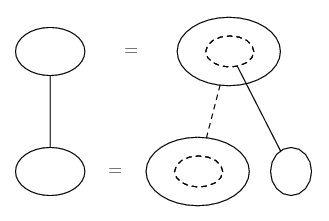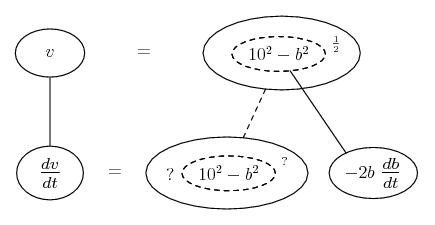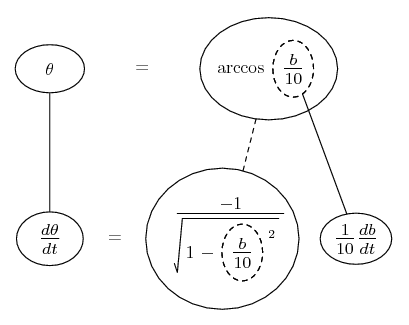# Thread: rate problem...

1. ## rate problem...

A ladder of length 10 meters is leaning against a vertical wall. if the base of the ladder is moving towards the wall at a rate of 2 meters/second, find following at the instant the base of the ladder is 7 meters from the wall.

a) how fast is the top of the ladder moving
b) the rate at which the angle between the ground and the ladder is changing

can anyone help me out please?

2. Just in case a picture helps...

Related rates nearly always depend on the chain rule, so you might want to try filling up this pattern...... where straight continuous lines differentiate downwards (integrate up) with respect to the main variable (in this case time), and the straight dashed line similarly but with respect to the dashed balloon expression (the inner function of the composite which is subject to the chain rule).

So what have we got here? b the base of the right triangle, 10 the length of the hypotenuse, v the vertical height, db/dt the (negative) rate of increase of b, and Pythagoras giving v in terms of b, as in the top row here...So differentiate with respect to the inner function (dashed balloon), then plug in the given values of b and db/dt.

Similar reasoning for the angle between base and hypotenuse...__________________________________________

Don't integrate - balloontegrate!

Balloon Calculus: Gallery

Balloon Calculus Drawing with LaTeX and Asymptote!

3. For part (a) think of the horizontal changes as changes in x and the vertical changes as y in a right triangle XYZ.

$\displaystyle x^2+y^2=z^2$

differentiate...
$\displaystyle 2x\frac{dx}{dt}+2y\frac{dy}{dt}=2z\frac{dz}{dt}$

Divide by two to ease up on the numbers $\displaystyle x\frac{dx}{dt}+y\frac{dy}{dt}=z\frac{dz}{dt}$

You know that $\displaystyle x=7$ because the ladder is 7 meters from the base of the wall. You know that $\displaystyle \frac{dx}{dt}=-2$ because the ladder is decreasing the gap between the wall and the ladder at 2 meters per sec. You know that $\displaystyle y=\sqrt{51}$ because of the Pythagorean theorem. Moreover you know that $\displaystyle \frac{dz}{dt}=0$ because z always equals 10.

Plug in those numbers and you will get $\displaystyle 7*-2+\sqrt{51}\frac{dy}{dt}=0$ From that, you get $\displaystyle \frac{dy}{dt}=\frac{14}{\sqrt{51}}$ meters per second.

4. For part (b), you are trying to find the rate of change of angle Y which i will refer to as $\displaystyle \frac{d\theta}{dt}$ from now on. To find $\displaystyle \frac{d\theta}{dt}$, you can use the equation $\displaystyle \cos{\theta}=\frac{x}{10}$.

Derive the equation to find $\displaystyle -\sin{\theta}\frac{d\theta}{dt}=\frac{\frac{dx}{dt} }{10}$

You know that x=7 and z=10 so $\displaystyle \sin{\theta}=\frac{\sqrt{51}}{10}$

So, $\displaystyle -\frac{\sqrt{51}}{10}\frac{d\theta}{dt}=\frac{1}{10 }\frac{dx}{dt}$

You already know from the problem that $\displaystyle \frac{dx}{dt}=-2$

Therefore, $\displaystyle \frac{d\theta}{dt}=-2\frac{1}{10}\frac{-10}{\sqrt{51}}=\frac{2}{\sqrt{51}}$ radians per second

5.Originally Posted by Keithfert488For part (a) think of the horizontal changes as changes in x and the vertical changes as y in a right triangle XYZ.

$\displaystyle x^2+y^2=z^2$

differentiate...
$\displaystyle 2x\frac{dx}{dt}+2y\frac{dy}{dt}=2z\frac{dz}{dt}$

Divide by two to ease up on the numbers $\displaystyle x\frac{dx}{dt}+y\frac{dy}{dt}=z\frac{dz}{dt}$

You know that $\displaystyle x=7$ because the ladder is 7 meters from the base of the wall. You know that $\displaystyle \frac{dx}{dt}=-2$ because the ladder is decreasing the gap between the wall and the ladder at 2 meters per sec. You know that $\displaystyle y=\sqrt{51}$ because of the Pythagorean theorem. Moreover you know that $\displaystyle \frac{dz}{dt}=0$ because z always equals 10.

Plug in those numbers and you will get $\displaystyle 7*-2+\sqrt{51}\frac{dy}{dt}=0$ From that, you get $\displaystyle \frac{dy}{dt}=\frac{14}{\sqrt{51}}$ meters per second.
wow. thats nice but using plain logic, wouldnt the ladder's vertical velocity = negative of ladders horizontal velocity thus vertical velocity = 2, whilst your answer gives $\displaystyle 7/sqrt(51)$ which isnt exactly 2?
thats confusing me, otherwise your method is correct

6.Originally Posted by Keithfert488You know that x=7 and z=10 so $\displaystyle \sin{\theta}=\frac{7}{10}$
Typo... this is cos so use Pythag to get sinOriginally Posted by purebladeknightwow. thats nice but using plain logic, wouldnt the ladder's vertical velocity = negative of ladders horizontal velocity thus vertical velocity = 2, whilst your answer gives $\displaystyle 7/sqrt(51)$ which isnt exactly 2?
thats confusing me, otherwise your method is correct
Granted vertical and horizontal have the same time to cover their distances but these distances are different except earlier when the ladder itself was horizontal!

7. Thanks for pointing out the typo. Is my answer correct now?

8. I'd be honoured if you were to compare it with mine...

(But yes it is.)

#### Search Tags

problem, rate